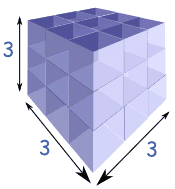Definition of

# Cube RootThe cube root of a number is a special value that, when used in a multiplication three times, gives that number.

Example: The cube root of 27 is 3 because 3 × 3 × 3 = 27.

Also the cube root of 64 is 4 because 4 × 4 × 4 = 64,
and so on.